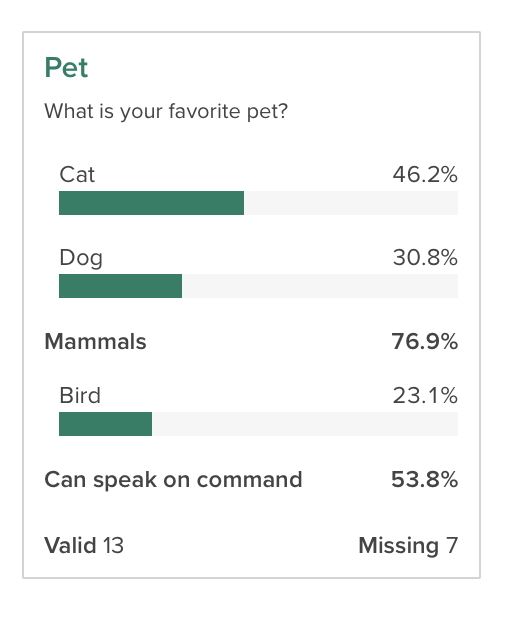library(crunch)

Previous: exporting data

A common task in the market research world is to collapse two or more categories together to see how the collapsed categories compare to one another. For example, if you asked people to rate their preference on a scale of 1 to 10, you might want to see how the people who provide a rating between 1 and 5 compare to those who rated it between 6 and 10. This goes by a number of names, including “Top Box” or “Nets”, depending on the use case. In Crunch, we call this family of features Subtotals. This vignette shows how to define, manage, and analyze variables with subtotals.

## Getting and setting subtotals on a categorical variable

Subtotals can be applied to any Categorical or Categorical Array variable. In R, we can view and set subtotal definitions with the subtotals() function. If there are no subtotals, the function will return NULL:

subtotals(ds$q1) ## NULL To add subtotals, we can assign a list of Subtotal objects. Each Subtotal object has three things: a name to identify it; a set of categories to pool together, referenced either by category name or id; and a location to show it, either after a given category or with position="top" or "bottom" to pin it first or last in the list. subtotals(ds$q1) <- list(
Subtotal(
name = "Mammals",
categories = c("Cat", "Dog"),
after = "Dog"
),
Subtotal(
name = "Can speak on command",
categories = c("Dog", "Bird"),
after = "Bird"
)
)

Now, if we check subtotals(), we can see that we have saved them. In this output we see a few different aspects of subtotals: the anchor is the id of the category to put the subtotal after (matching the after or position argument in Subtotal()), name, aggregation functions and args, which in the this case are the category ids to include in the subtotal.

subtotals(ds\$q1)
##   anchor                 name     func    args               kwargs
## 1      2              Mammals subtotal 1 and 2 positive: 1 and 2 |
## 2      3 Can speak on command subtotal 2 and 3 positive: 2 and 3 |

This shows up in the Categorical variable card on the web app like this:Crunch also supports “Subtotal Differences” (sometimes also called “Net Promoter Scores”